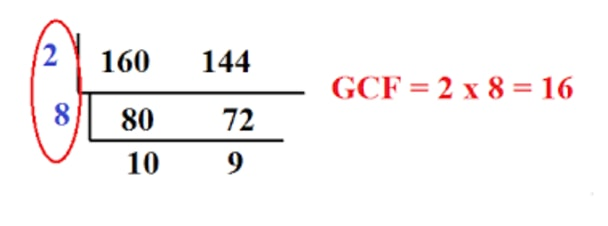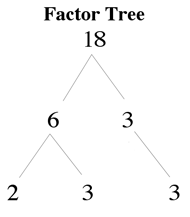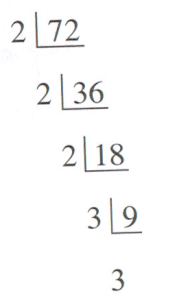# Prime factorization#### Everything You Need in One Place

Homework problems? Exam preparation? Trying to grasp a concept or just brushing up the basics? Our extensive help & practice library have got you covered.#### Learn and Practise With Ease

Our proven video lessons ease you through problems quickly, and you get tonnes of friendly practise on questions that trip students up on tests and finals.#### Instant and Unlimited Help

Our personalized learning platform enables you to instantly find the exact walkthrough to your specific type of question. Activate unlimited help now!

0/1
##### Intros
###### Lessons
1. What is prime factorization?
0/6
##### Examples
###### Lessons
1. Determine if the numbers are prime or composite.
1. 19, 199, 118
2. What are the prime factors of the following numbers?
1. 48
2. 525
3. 100
4. 4550
5. 2318
0%
##### Practice
###### Free to Join!
StudyPug is a learning help platform covering math and science from grade 4 all the way to second year university. Our video tutorials, unlimited practice problems, and step-by-step explanations provide you or your child with all the help you need to master concepts. On top of that, it's fun - with achievements, customizable avatars, and awards to keep you motivated.
• #### Easily See Your ProgressWe track the progress you've made on a topic so you know what you've done. From the course view you can easily see what topics have what and the progress you've made on them. Fill the rings to completely master that section or mouse over the icon to see more details.
• #### Make Use of Our Learning Aids###### Practice Accuracy

See how well your practice sessions are going over time.

Stay on track with our daily recommendations.

• #### Earn Achievements as You LearnMake the most of your time as you use StudyPug to help you achieve your goals. Earn fun little badges the more you watch, practice, and use our service.
• #### Create and Customize Your AvatarPlay with our fun little avatar builder to create and customize your own avatar on StudyPug. Choose your face, eye colour, hair colour and style, and background. Unlock more options the more you use StudyPug.
###### Topic Notes
A prime number is a whole number which can only be divided evenly by one or itself. Composite numbers are whole numbers that is not prime or has more than two factors. Prime factorization is a process that looks for prime factors of a number.

## Factor

One of the first things you'll need to learn about before tackling prime and composite numbers are factors. Factors are what you'll have to multiply together in order to get a certain number. So for example, the factors of 18 is 2 and 9 since 2 x 9 = 18. The divisor definition is a number that another number is to be divided with. In this case, 2 and 9 are each also known as a divisor.

## Prime numbers

So what are prime numbers? Prime numbers are numbers that can only be factored by 1 and itself. An example of this is 2. You cannot get an answer of 2 in multiplication other than multiplying 2 with 1. 2 is known as a prime divisor.

## Composite Numbers

Contrary to prime numbers, composite numbers are numbers that have more factors than just 1 and itself. It also is positive. For example, 9 can be factored into 3 x 3 or it can be 9 x 1. All whole numbers are either prime or composite, other than the number 1 and 0. 0 has an infinite amount of factors, whereas 1 cannot be made up of anything that is not itself.

## Factor Tree

To tackle the questions in this lesson, you're going to have to learn about a factor tree. A factor tree breaks down a number so that you're able to identify its prime factors. The steps to making a factor tree is:

1) Write down the number you are trying to factorize at the top of the tree

2) Draw two branches stemming from the number downwards

3) Break down the original number into two factors and write it at the end of the branches you just drew in the previous step

4) Continue breaking down the numbers into factors at the end of branches until you're left with all prime numbers and there are no more factors to be found

5) Take all the numbers at the end of the factor tree branches to find out the prime factors of your original number

## Continuous division

You can use a method called continuous division to find the greatest common factor (GCF) of a number. The GCF also deals with prime numbers. You can carry out continuous division by:

1) Writing down the two numbers you're trying to find the GCF of

2) Draw an "L" shape surrounding them

3) Divide both the numbers by a common factor

4) Write the answers you get underneath the bar

5) Continue doing this until all the numbers you're left with as answers are prime numbers

6) Multiply together all the numbers on the left hand side (that has been common factors of the numbers inside the "L") and you'll get your GCF

## Example problems

Question 1:

1a) Factors of $12$

Solution:

$12$

$4 \bullet 3$

Since $4$ is not a prime number, we can break it down into two times two

$2 \bullet 2 \bullet 3$

We are done now because all the numbers are prime, but we can group up the same numbers in exponent form.

$2^{2} \bullet 3$

1b) Factors of $24$

Solution:

$24$

$2 \bullet 12$

$2 \bullet 2 \bullet 6$

$2 \bullet 2 \bullet 2 \bullet 3$

And in exponent form

$2^{3}\bullet 3$

Question 2

2a) Factors $30$ using factor tree

Solution:

2b) Factors of $54$ using factor tree

Solution:

2c) Factors of $28$, using tree

Solution:

$14$ is not prime, so it can be factored further.

Question 3

What is the greatest common factor between $160$ and $144$, using continuous division?Use continuous division to find greatest common factor of two numbers

Got a number in mind you wanted to check the prime factors for? Here's a prime factor calculator you can check out.

Want to learn more related to this lesson? Take a look at how to use exponents to describe numbers, the product rule of exponents, how to find common factors of polynomials, and factoring polynomials.

Factor:
Numbers that can be multiplied together to give the original value.
Ex: The factor of 15 is 5 & 3. Because $5 \times 3 = 15$
Prime numbers:
A whole number that has only "two" factors: 1 & itself.
Ex: 2,3,5,7,11,13,17…
Composite numbers:
A Whole number that is not prime or has more than 2 factors.
Ex: 4,6,8…
Zero & One:
They are neither prime nor composite.
Factor Tree:
A method of finding prime factors by using a tree diagram.
Ex: Prime factors of $18 = 2 \times 3 \times 3$Continuous Division:
A method of finding prime factors by using a continuous division.
Ex: Prime factors of $72 = 2 \times 2 \times 2 \times 3 \times 3$